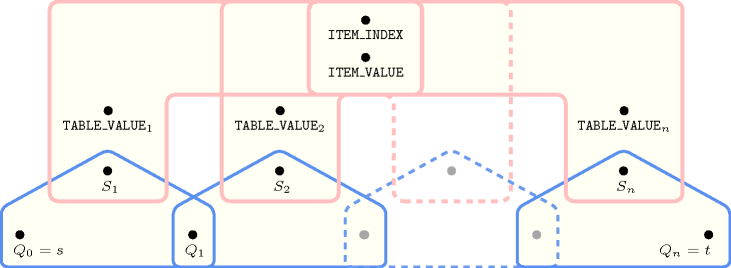## 5.137. elem

Origin
Constraint

$\mathrm{𝚎𝚕𝚎𝚖}\left(\mathrm{𝙸𝚃𝙴𝙼},\mathrm{𝚃𝙰𝙱𝙻𝙴}\right)$

Usual name

$\mathrm{𝚎𝚕𝚎𝚖𝚎𝚗𝚝}$

Synonyms

$\mathrm{𝚗𝚝𝚑}$, $\mathrm{𝚊𝚛𝚛𝚊𝚢}$.

Arguments
 $\mathrm{𝙸𝚃𝙴𝙼}$ $\mathrm{𝚌𝚘𝚕𝚕𝚎𝚌𝚝𝚒𝚘𝚗}\left(\mathrm{𝚒𝚗𝚍𝚎𝚡}-\mathrm{𝚍𝚟𝚊𝚛},\mathrm{𝚟𝚊𝚕𝚞𝚎}-\mathrm{𝚍𝚟𝚊𝚛}\right)$ $\mathrm{𝚃𝙰𝙱𝙻𝙴}$ $\mathrm{𝚌𝚘𝚕𝚕𝚎𝚌𝚝𝚒𝚘𝚗}\left(\mathrm{𝚒𝚗𝚍𝚎𝚡}-\mathrm{𝚒𝚗𝚝},\mathrm{𝚟𝚊𝚕𝚞𝚎}-\mathrm{𝚍𝚟𝚊𝚛}\right)$
Restrictions
 $\mathrm{𝚛𝚎𝚚𝚞𝚒𝚛𝚎𝚍}$$\left(\mathrm{𝙸𝚃𝙴𝙼},\left[\mathrm{𝚒𝚗𝚍𝚎𝚡},\mathrm{𝚟𝚊𝚕𝚞𝚎}\right]\right)$ $\mathrm{𝙸𝚃𝙴𝙼}.\mathrm{𝚒𝚗𝚍𝚎𝚡}\ge 1$ $\mathrm{𝙸𝚃𝙴𝙼}.\mathrm{𝚒𝚗𝚍𝚎𝚡}\le |\mathrm{𝚃𝙰𝙱𝙻𝙴}|$ $|\mathrm{𝙸𝚃𝙴𝙼}|=1$ $|\mathrm{𝚃𝙰𝙱𝙻𝙴}|>0$ $\mathrm{𝚛𝚎𝚚𝚞𝚒𝚛𝚎𝚍}$$\left(\mathrm{𝚃𝙰𝙱𝙻𝙴},\left[\mathrm{𝚒𝚗𝚍𝚎𝚡},\mathrm{𝚟𝚊𝚕𝚞𝚎}\right]\right)$ $\mathrm{𝚃𝙰𝙱𝙻𝙴}.\mathrm{𝚒𝚗𝚍𝚎𝚡}\ge 1$ $\mathrm{𝚃𝙰𝙱𝙻𝙴}.\mathrm{𝚒𝚗𝚍𝚎𝚡}\le |\mathrm{𝚃𝙰𝙱𝙻𝙴}|$ $\mathrm{𝚍𝚒𝚜𝚝𝚒𝚗𝚌𝚝}$$\left(\mathrm{𝚃𝙰𝙱𝙻𝙴},\mathrm{𝚒𝚗𝚍𝚎𝚡}\right)$
Purpose

$\mathrm{𝙸𝚃𝙴𝙼}$ is equal to one of the entries of the table $\mathrm{𝚃𝙰𝙱𝙻𝙴}$.

Example
$\left(\begin{array}{c}〈\mathrm{𝚒𝚗𝚍𝚎𝚡}-3\mathrm{𝚟𝚊𝚕𝚞𝚎}-2〉,\hfill \\ 〈\begin{array}{cc}\mathrm{𝚒𝚗𝚍𝚎𝚡}-1\hfill & \mathrm{𝚟𝚊𝚕𝚞𝚎}-6,\hfill \\ \mathrm{𝚒𝚗𝚍𝚎𝚡}-2\hfill & \mathrm{𝚟𝚊𝚕𝚞𝚎}-9,\hfill \\ \mathrm{𝚒𝚗𝚍𝚎𝚡}-3\hfill & \mathrm{𝚟𝚊𝚕𝚞𝚎}-2,\hfill \\ \mathrm{𝚒𝚗𝚍𝚎𝚡}-4\hfill & \mathrm{𝚟𝚊𝚕𝚞𝚎}-9\hfill \end{array}〉\hfill \end{array}\right)$

The $\mathrm{𝚎𝚕𝚎𝚖}$ constraint holds since its first argument $\mathrm{𝙸𝚃𝙴𝙼}$ corresponds to the third item of the $\mathrm{𝚃𝙰𝙱𝙻𝙴}$ collection.

Typical
 $|\mathrm{𝚃𝙰𝙱𝙻𝙴}|>1$ $\mathrm{𝚛𝚊𝚗𝚐𝚎}$$\left(\mathrm{𝚃𝙰𝙱𝙻𝙴}.\mathrm{𝚟𝚊𝚕𝚞𝚎}\right)>1$
Symmetries
• Items of $\mathrm{𝚃𝙰𝙱𝙻𝙴}$ are permutable.

• All occurrences of two distinct values in $\mathrm{𝙸𝚃𝙴𝙼}.\mathrm{𝚟𝚊𝚕𝚞𝚎}$ or $\mathrm{𝚃𝙰𝙱𝙻𝙴}.\mathrm{𝚟𝚊𝚕𝚞𝚎}$ can be swapped; all occurrences of a value in $\mathrm{𝙸𝚃𝙴𝙼}.\mathrm{𝚟𝚊𝚕𝚞𝚎}$ or $\mathrm{𝚃𝙰𝙱𝙻𝙴}.\mathrm{𝚟𝚊𝚕𝚞𝚎}$ can be renamed to any unused value.

Arg. properties

Functional dependency: $\mathrm{𝙸𝚃𝙴𝙼}.\mathrm{𝚟𝚊𝚕𝚞𝚎}$ determined by $\mathrm{𝙸𝚃𝙴𝙼}.\mathrm{𝚒𝚗𝚍𝚎𝚡}$ and $\mathrm{𝚃𝙰𝙱𝙻𝙴}$.

Usage

Makes the link between the discrete decision variable $\mathrm{𝙸𝙽𝙳𝙴𝚇}$ and the variable $\mathrm{𝚅𝙰𝙻𝚄𝙴}$ according to a given table of values $\mathrm{𝚃𝙰𝙱𝙻𝙴}$. We now give five typical uses of the $\mathrm{𝚎𝚕𝚎𝚖}$ constraint.

1. In some problems we may have to represent a function $y=f\left(x\right)$ (with $x\in \left[1,m\right]$). In this context we generate the following $\mathrm{𝚎𝚕𝚎𝚖}$ constraint where $\mathrm{𝙸𝙽𝙳𝙴𝚇}$ is a domain variable taking its values in $\left\{1,2,\cdots ,m\right\}$:

$\mathrm{𝚎𝚕𝚎𝚖}\left(\begin{array}{c}〈\begin{array}{cc}\mathrm{𝚒𝚗𝚍𝚎𝚡}-x\hfill & \mathrm{𝚟𝚊𝚕𝚞𝚎}-y\hfill \end{array}〉,\hfill \\ 〈\begin{array}{cc}\mathrm{𝚒𝚗𝚍𝚎𝚡}-1\hfill & \mathrm{𝚟𝚊𝚕𝚞𝚎}-f\left(1\right),\hfill \\ \mathrm{𝚒𝚗𝚍𝚎𝚡}-2\hfill & \mathrm{𝚟𝚊𝚕𝚞𝚎}-f\left(2\right),\hfill \\ & ⋮\hfill \\ \mathrm{𝚒𝚗𝚍𝚎𝚡}-m\hfill & \mathrm{𝚟𝚊𝚕𝚞𝚎}-f\left(m\right)\hfill \end{array}〉\hfill \end{array}\right)$
##### Figure 5.137.1. $y={x}^{3}$ ($1\le x\le 3$)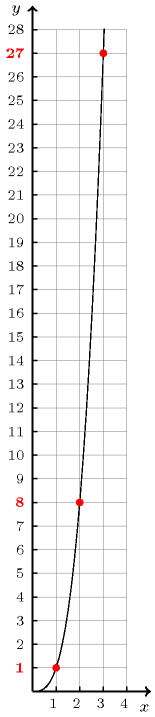As an example, consider the problem of finding the smallest integer that can be decomposed in two different ways in the sum of two cubes [HardyWright75]. The $\mathrm{𝚎𝚕𝚎𝚖}$ constraint can be used for representing the function $y={x}^{3}$ (Figure 5.137.1). The unique solution $1729={12}^{3}+{1}^{3}={10}^{3}+{9}^{3}$ can be obtained by the following set of constraints:

$\left\{\begin{array}{c}\mathrm{𝚎𝚕𝚎𝚖}\left(〈\mathrm{𝚒𝚗𝚍𝚎𝚡}-{x}_{1}\mathrm{𝚟𝚊𝚕𝚞𝚎}-{y}_{1}〉,\hfill \\ 〈\mathrm{𝚒𝚗𝚍𝚎𝚡}-1\mathrm{𝚟𝚊𝚕𝚞𝚎}-1,\mathrm{𝚒𝚗𝚍𝚎𝚡}-2\mathrm{𝚟𝚊𝚕𝚞𝚎}-8,\cdots ,\mathrm{𝚒𝚗𝚍𝚎𝚡}-20\mathrm{𝚟𝚊𝚕𝚞𝚎}-8000〉\right)\hfill \\ \mathrm{𝚎𝚕𝚎𝚖}\left(〈\mathrm{𝚒𝚗𝚍𝚎𝚡}-{x}_{2}\mathrm{𝚟𝚊𝚕𝚞𝚎}-{y}_{2}〉,\hfill \\ 〈\mathrm{𝚒𝚗𝚍𝚎𝚡}-1\mathrm{𝚟𝚊𝚕𝚞𝚎}-1,\mathrm{𝚒𝚗𝚍𝚎𝚡}-2\mathrm{𝚟𝚊𝚕𝚞𝚎}-8,\cdots ,\mathrm{𝚒𝚗𝚍𝚎𝚡}-20\mathrm{𝚟𝚊𝚕𝚞𝚎}-8000〉\right)\hfill \\ \mathrm{𝚎𝚕𝚎𝚖}\left(〈\mathrm{𝚒𝚗𝚍𝚎𝚡}-{x}_{3}\mathrm{𝚟𝚊𝚕𝚞𝚎}-{y}_{3}〉,\hfill \\ 〈\mathrm{𝚒𝚗𝚍𝚎𝚡}-1\mathrm{𝚟𝚊𝚕𝚞𝚎}-1,\mathrm{𝚒𝚗𝚍𝚎𝚡}-2\mathrm{𝚟𝚊𝚕𝚞𝚎}-8,\cdots ,\mathrm{𝚒𝚗𝚍𝚎𝚡}-20\mathrm{𝚟𝚊𝚕𝚞𝚎}-8000〉\right)\hfill \\ \mathrm{𝚎𝚕𝚎𝚖}\left(〈\mathrm{𝚒𝚗𝚍𝚎𝚡}-{x}_{4}\mathrm{𝚟𝚊𝚕𝚞𝚎}-{y}_{4}〉,\hfill \\ 〈\mathrm{𝚒𝚗𝚍𝚎𝚡}-1\mathrm{𝚟𝚊𝚕𝚞𝚎}-1,\mathrm{𝚒𝚗𝚍𝚎𝚡}-2\mathrm{𝚟𝚊𝚕𝚞𝚎}-8,\cdots ,\mathrm{𝚒𝚗𝚍𝚎𝚡}-20\mathrm{𝚟𝚊𝚕𝚞𝚎}-8000〉\right)\hfill \\ {y}_{1}+{y}_{2}={y}_{3}+{y}_{4}\hfill \\ {x}_{1}<{x}_{2}\hfill \\ {x}_{3}<{x}_{4}\hfill \\ {x}_{1}<{x}_{3}\hfill \end{array}\right\$ The last three inequalities constraints in the conjunction are used for breaking symmetries. The constraints ${x}_{1}<{x}_{2}$ and ${x}_{3}<{x}_{4}$ respectively order the pairs of variables $\left({x}_{1},{x}_{2}\right)$ and $\left({x}_{3},{x}_{4}\right)$ from which the sums ${x}_{1}^{3}+{x}_{2}^{3}$ and ${x}_{3}^{3}+{x}_{4}^{3}$ are generated. Finally the inequality ${x}_{1}<{x}_{3}$ enforces a lexicographic ordering between the two pairs of variables $\left({x}_{1},{x}_{2}\right)$ and $\left({x}_{3},{x}_{4}\right)$.

2. In some optimisation problems a classical use of the $\mathrm{𝚎𝚕𝚎𝚖}$ constraint consists expressing the link between a discrete choice and its corresponding cost. For each discrete choice we create an $\mathrm{𝚎𝚕𝚎𝚖}$ constraint of the form:

$\mathrm{𝚎𝚕𝚎𝚖}\left(\begin{array}{c}〈\begin{array}{cc}\mathrm{𝚒𝚗𝚍𝚎𝚡}-\mathrm{𝙲𝚑𝚘𝚒𝚌𝚎}\hfill & \mathrm{𝚟𝚊𝚕𝚞𝚎}-\mathrm{𝙲𝚘𝚜𝚝}\hfill \end{array}〉,\hfill \\ 〈\begin{array}{cc}\mathrm{𝚒𝚗𝚍𝚎𝚡}-1\hfill & \mathrm{𝚟𝚊𝚕𝚞𝚎}-{\mathrm{𝙲𝚘𝚜𝚝}}_{1},\hfill \\ \mathrm{𝚒𝚗𝚍𝚎𝚡}-2\hfill & \mathrm{𝚟𝚊𝚕𝚞𝚎}-{\mathrm{𝙲𝚘𝚜𝚝}}_{2},\hfill \\ & ⋮\hfill \\ \mathrm{𝚒𝚗𝚍𝚎𝚡}-m\hfill & \mathrm{𝚟𝚊𝚕𝚞𝚎}-{\mathrm{𝙲𝚘𝚜𝚝}}_{m}\hfill \end{array}〉\hfill \end{array}\right)$

where:

• $\mathrm{𝙲𝚑𝚘𝚒𝚌𝚎}$ is a domain variable that indicates which alternative will be finally selected,

• $\mathrm{𝙲𝚘𝚜𝚝}$ is a domain variable that corresponds to the cost of the decision associated with the value of the $\mathrm{𝙲𝚑𝚘𝚒𝚌𝚎}$ variable,

• ${\mathrm{𝙲𝚘𝚜𝚝}}_{1},{\mathrm{𝙲𝚘𝚜𝚝}}_{2},\cdots ,{\mathrm{𝙲𝚘𝚜𝚝}}_{m}$ are the respective costs associated with the alternatives $1,2,\cdots ,m$.

3. In some problems we need to express a disjunction of the form $\mathrm{𝚅𝙰𝚁}={\mathrm{𝚅𝙰𝚁}}_{1}\vee \mathrm{𝚅𝙰𝚁}={\mathrm{𝚅𝙰𝚁}}_{2}\vee \cdots \vee \mathrm{𝚅𝙰𝚁}={\mathrm{𝚅𝙰𝚁}}_{n}$. This can be directly reformulated as the following $\mathrm{𝚎𝚕𝚎𝚖}$ constraint, where $\mathrm{𝙸𝙽𝙳𝙴𝚇}$ is a domain variable taking its value in the finite set $\left\{1,2,\cdots ,n\right\}$ and where the $\mathrm{𝚃𝙰𝙱𝙻𝙴}$ argument corresponds to the domain variables ${\mathrm{𝚅𝙰𝚁}}_{1},{\mathrm{𝚅𝙰𝚁}}_{2},\cdots ,{\mathrm{𝚅𝙰𝚁}}_{n}$:

$\mathrm{𝚎𝚕𝚎𝚖}\left(\begin{array}{c}〈\begin{array}{cc}\mathrm{𝚒𝚗𝚍𝚎𝚡}-\mathrm{𝙸𝙽𝙳𝙴𝚇}\hfill & \mathrm{𝚟𝚊𝚕𝚞𝚎}-\mathrm{𝚅𝙰𝚁}\hfill \end{array}〉,\hfill \\ 〈\begin{array}{cc}\mathrm{𝚒𝚗𝚍𝚎𝚡}-1\hfill & \mathrm{𝚟𝚊𝚕𝚞𝚎}-{\mathrm{𝚅𝙰𝚁}}_{1},\hfill \\ \mathrm{𝚒𝚗𝚍𝚎𝚡}-2\hfill & \mathrm{𝚟𝚊𝚕𝚞𝚎}-{\mathrm{𝚅𝙰𝚁}}_{2},\hfill \\ & ⋮\hfill \\ \mathrm{𝚒𝚗𝚍𝚎𝚡}-n\hfill & \mathrm{𝚟𝚊𝚕𝚞𝚎}-{\mathrm{𝚅𝙰𝚁}}_{n}\hfill \end{array}〉\hfill \end{array}\right)$
4. In some scheduling problems the duration of a task depends on the machine where the task will be assigned in final schedule. In this case we generate for each task an $\mathrm{𝚎𝚕𝚎𝚖}$ constraint of the following form:

$\mathrm{𝚎𝚕𝚎𝚖}\left(\begin{array}{c}〈\begin{array}{cc}\mathrm{𝚒𝚗𝚍𝚎𝚡}-\mathrm{𝙼𝚊𝚌𝚑𝚒𝚗𝚎}\hfill & \mathrm{𝚟𝚊𝚕𝚞𝚎}-\mathrm{𝙳𝚞𝚛𝚊𝚝𝚒𝚘𝚗}\hfill \end{array}〉,\hfill \\ 〈\begin{array}{cc}\mathrm{𝚒𝚗𝚍𝚎𝚡}-1\hfill & \mathrm{𝚟𝚊𝚕𝚞𝚎}-{\mathrm{𝙳𝚞𝚛}}_{1},\hfill \\ \mathrm{𝚒𝚗𝚍𝚎𝚡}-2\hfill & \mathrm{𝚟𝚊𝚕𝚞𝚎}-{\mathrm{𝙳𝚞𝚛}}_{2},\hfill \\ & ⋮\hfill \\ \mathrm{𝚒𝚗𝚍𝚎𝚡}-m\hfill & \mathrm{𝚟𝚊𝚕𝚞𝚎}-{\mathrm{𝙳𝚞𝚛}}_{m}\hfill \end{array}〉\hfill \end{array}\right)$

where:

• $\mathrm{𝙼𝚊𝚌𝚑𝚒𝚗𝚎}$ is a domain variable that indicates the resource to which the task will be assigned,

• $\mathrm{𝙳𝚞𝚛𝚊𝚝𝚒𝚘𝚗}$ is a domain variable that corresponds to the duration of the task,

• ${\mathrm{𝙳𝚞𝚛}}_{1},{\mathrm{𝙳𝚞𝚛}}_{2},\cdots ,{\mathrm{𝙳𝚞𝚛}}_{m}$ are the respective duration of the task according to the hypothesis that it runs on machine $1,2$ or $m$.

##### Figure 5.137.2. A task $t$ for which the duration depends on the machine 1, 2 or 3 to which it is assigned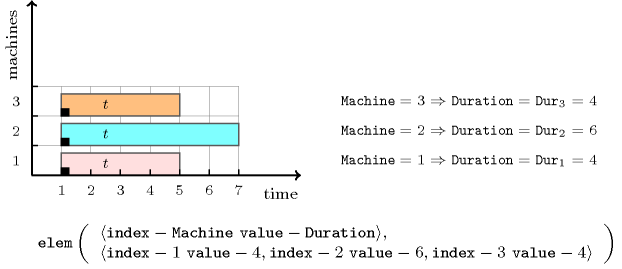Figure 5.137.2 illustrates this particular use of the $\mathrm{𝚎𝚕𝚎𝚖}$ constraint for modelling that a task has a duration of 4, 6 and 4 when we respectively assign it on machines 1, 2 and 3.

5. In some vehicle routing problems we typically use the $\mathrm{𝚎𝚕𝚎𝚖}$ constraint to express the distance between location $i$ and the next location visited by a vehicle. For this purpose we generate for each location $i$ an $\mathrm{𝚎𝚕𝚎𝚖}$ constraint of the form:

$\mathrm{𝚎𝚕𝚎𝚖}\left(\begin{array}{c}〈\begin{array}{cc}\mathrm{𝚒𝚗𝚍𝚎𝚡}-{\mathrm{𝙽𝚎𝚡𝚝}}_{i}\hfill & \mathrm{𝚟𝚊𝚕𝚞𝚎}-{\mathrm{𝚍𝚒𝚜𝚝𝚊𝚗𝚌𝚎}}_{i}\hfill \end{array}〉,\hfill \\ 〈\begin{array}{cc}\mathrm{𝚒𝚗𝚍𝚎𝚡}-1\hfill & \mathrm{𝚟𝚊𝚕𝚞𝚎}-{\mathrm{𝙳𝚒𝚜𝚝}}_{{i}_{1}},\hfill \\ \mathrm{𝚒𝚗𝚍𝚎𝚡}-2\hfill & \mathrm{𝚟𝚊𝚕𝚞𝚎}-{\mathrm{𝙳𝚒𝚜𝚝}}_{{i}_{2}},\hfill \\ & ⋮\hfill \\ \mathrm{𝚒𝚗𝚍𝚎𝚡}-m\hfill & \mathrm{𝚟𝚊𝚕𝚞𝚎}-{\mathrm{𝙳𝚒𝚜𝚝}}_{{i}_{m}}\hfill \end{array}〉\hfill \end{array}\right)$

where:

• ${\mathrm{𝙽𝚎𝚡𝚝}}_{i}$ is a domain variable that gives the index of the location the vehicle will visit just after location $i$,

• ${\mathrm{𝚍𝚒𝚜𝚝𝚊𝚗𝚌𝚎}}_{i}$ is a domain variable that corresponds to the distance between location $i$ and the location the vehicle will visit just after,

• ${\mathrm{𝙳𝚒𝚜𝚝}}_{{i}_{1}},{\mathrm{𝙳𝚒𝚜𝚝}}_{{i}_{2}},\cdots ,{\mathrm{𝙳𝚒𝚜𝚝}}_{{i}_{m}}$ are the respective distances between location $i$ and locations $1,2,\cdots ,m$.

An other example where the table argument corresponds to domain variables is described in the keyword entry assignment to the same set of values.

Remark

Originally, the parameters of the $\mathrm{𝚎𝚕𝚎𝚖}$ constraint had the form $\mathrm{𝚎𝚕𝚎𝚖𝚎𝚗𝚝}\left(\mathrm{𝙸𝙽𝙳𝙴𝚇},\mathrm{𝚃𝙰𝙱𝙻𝙴},\mathrm{𝚅𝙰𝙻𝚄𝙴}\right)$, where $\mathrm{𝙸𝙽𝙳𝙴𝚇}$ and $\mathrm{𝚅𝙰𝙻𝚄𝙴}$ were two domain variables and $\mathrm{𝚃𝙰𝙱𝙻𝙴}$ was a list of non-negative integers.

Within some systems (e.g., Gecode), the index of the first entry of the table $\mathrm{𝚃𝙰𝙱𝙻𝙴}$ corresponds to 0 rather than to 1.

When the first entry of the table $\mathrm{𝚃𝙰𝙱𝙻𝙴}$ corresponds to a value $p$ that is different from 1 we can still use the $\mathrm{𝚎𝚕𝚎𝚖}$ constraint. We use the reformulation $I=J-p+1$ $\wedge$ $\mathrm{𝚎𝚕𝚎𝚖}\left(〈\mathrm{𝚒𝚗𝚍𝚎𝚡}-I\mathrm{𝚟𝚊𝚕𝚞𝚎}-V〉,\mathrm{𝚃𝙰𝙱𝙻𝙴}\right)$, where $I$ and $J$ are domain variables respectively ranging from 1 to $|\mathrm{𝚃𝙰𝙱𝙻𝙴}|$ and from $p$ to $p+|\mathrm{𝚃𝙰𝙱𝙻𝙴}|-1$.

Systems

nth in Choco, element in Gecode, element in JaCoP, element in SICStus.

Keywords
Cond. implications

$\mathrm{𝚎𝚕𝚎𝚖}\left(\mathrm{𝙸𝚃𝙴𝙼},\mathrm{𝚃𝙰𝙱𝙻𝙴}\right)$

with  $\mathrm{𝚃𝙰𝙱𝙻𝙴}.\mathrm{𝚟𝚊𝚕𝚞𝚎}\ge 0$

implies $\mathrm{𝚋𝚒𝚗}_\mathrm{𝚙𝚊𝚌𝚔𝚒𝚗𝚐}_\mathrm{𝚌𝚊𝚙𝚊}$$\left(\mathrm{𝚃𝙰𝙱𝙻𝙴},\mathrm{𝙸𝚃𝙴𝙼}\right)$.

Arc input(s)

$\mathrm{𝙸𝚃𝙴𝙼}$ $\mathrm{𝚃𝙰𝙱𝙻𝙴}$

Arc generator
$\mathrm{𝑃𝑅𝑂𝐷𝑈𝐶𝑇}$$↦\mathrm{𝚌𝚘𝚕𝚕𝚎𝚌𝚝𝚒𝚘𝚗}\left(\mathrm{𝚒𝚝𝚎𝚖},\mathrm{𝚝𝚊𝚋𝚕𝚎}\right)$

Arc arity
Arc constraint(s)
 $•\mathrm{𝚒𝚝𝚎𝚖}.\mathrm{𝚒𝚗𝚍𝚎𝚡}=\mathrm{𝚝𝚊𝚋𝚕𝚎}.\mathrm{𝚒𝚗𝚍𝚎𝚡}$ $•\mathrm{𝚒𝚝𝚎𝚖}.\mathrm{𝚟𝚊𝚕𝚞𝚎}=\mathrm{𝚝𝚊𝚋𝚕𝚎}.\mathrm{𝚟𝚊𝚕𝚞𝚎}$
Graph property(ies)
$\mathrm{𝐍𝐀𝐑𝐂}$$=1$

Graph model

We regroup the $\mathrm{𝙸𝙽𝙳𝙴𝚇}$ and $\mathrm{𝚅𝙰𝙻𝚄𝙴}$ parameters of the original $\mathrm{𝚎𝚕𝚎𝚖𝚎𝚗𝚝}$ constraint $\mathrm{𝚎𝚕𝚎𝚖𝚎𝚗𝚝}\left(\mathrm{𝙸𝙽𝙳𝙴𝚇},\mathrm{𝚃𝙰𝙱𝙻𝙴},\mathrm{𝚅𝙰𝙻𝚄𝙴}\right)$ into the parameter $\mathrm{𝙸𝚃𝙴𝙼}$. We also make explicit the different indices of the table $\mathrm{𝚃𝙰𝙱𝙻𝙴}$.

Parts (A) and (B) of Figure 5.137.3 respectively show the initial and final graph associated with the Example slot. Since we use the $\mathrm{𝐍𝐀𝐑𝐂}$ graph property, the unique arc of the final graph is stressed in bold.

##### Figure 5.137.3. Initial and final graph of the $\mathrm{𝚎𝚕𝚎𝚖}$ constraint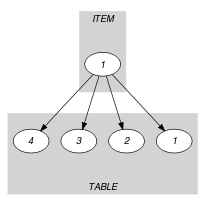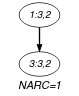(a) (b)
Signature

Since all the $\mathrm{𝚒𝚗𝚍𝚎𝚡}$ attributes of $\mathrm{𝚃𝙰𝙱𝙻𝙴}$ are distinct and because of the first condition of the arc constraint the final graph cannot have more than one arc. Therefore we can rewrite $\mathrm{𝐍𝐀𝐑𝐂}=1$ to $\mathrm{𝐍𝐀𝐑𝐂}\ge 1$ and simplify $\underline{\overline{\mathrm{𝐍𝐀𝐑𝐂}}}$ to $\overline{\mathrm{𝐍𝐀𝐑𝐂}}$.

Automaton

Figure 5.137.4 depicts the automaton associated with the $\mathrm{𝚎𝚕𝚎𝚖}$ constraint. Let $\mathrm{𝙸𝙽𝙳𝙴𝚇}$ and $\mathrm{𝚅𝙰𝙻𝚄𝙴}$ respectively be the $\mathrm{𝚒𝚗𝚍𝚎𝚡}$ and the $\mathrm{𝚟𝚊𝚕𝚞𝚎}$ attributes of the unique item of the $\mathrm{𝙸𝚃𝙴𝙼}$ collection. Let ${\mathrm{𝙸𝙽𝙳𝙴𝚇}}_{i}$ and ${\mathrm{𝚅𝙰𝙻𝚄𝙴}}_{i}$ respectively be the $\mathrm{𝚒𝚗𝚍𝚎𝚡}$ and the $\mathrm{𝚟𝚊𝚕𝚞𝚎}$ attributes of item $i$ of the $\mathrm{𝚃𝙰𝙱𝙻𝙴}$ collection. To each quadruple $\left(\mathrm{𝙸𝙽𝙳𝙴𝚇},\mathrm{𝚅𝙰𝙻𝚄𝙴},{\mathrm{𝙸𝙽𝙳𝙴𝚇}}_{i},{\mathrm{𝚅𝙰𝙻𝚄𝙴}}_{i}\right)$ corresponds a 0-1 signature variable ${S}_{i}$ as well as the following signature constraint: $\left(\left(\mathrm{𝙸𝙽𝙳𝙴𝚇}={\mathrm{𝙸𝙽𝙳𝙴𝚇}}_{i}\right)\wedge \left(\mathrm{𝚅𝙰𝙻𝚄𝙴}={\mathrm{𝚅𝙰𝙻𝚄𝙴}}_{i}\right)\right)⇔{S}_{i}$.

##### Figure 5.137.4. Automaton of the $\mathrm{𝚎𝚕𝚎𝚖}$$\left(\mathrm{𝙸𝚃𝙴𝙼},\mathrm{𝚃𝙰𝙱𝙻𝙴}\right)$ constraint (once one finds the right item – index and value – in the table, one switches from the initial state $s$ to the accepting state $t$)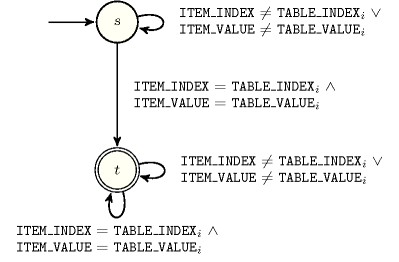##### Figure 5.137.5. Hypergraph of the reformulation corresponding to the automaton of the $\mathrm{𝚎𝚕𝚎𝚖}$ constraint